# Class A BJT Amplifiers

## Discrete Semiconductor Devices and Circuits

• #### Question 1

 Don’t just sit there! Build something!!

Learning to mathematically analyze circuits requires much study and practice. Typically, students practice by working through lots of sample problems and checking their answers against those provided by the textbook or the instructor. While this is good, there is a much better way.

You will learn much more by actually building and analyzing real circuits, letting your test equipment provide the “answers” instead of a book or another person. For successful circuit-building exercises, follow these steps:

1. Carefully measure and record all component values prior to circuit construction, choosing resistor values high enough to make damage to any active components unlikely.
2. Draw the schematic diagram for the circuit to be analyzed.
3. Carefully build this circuit on a breadboard or other convenient medium.
4. Check the accuracy of the circuit’s construction, following each wire to each connection point, and verifying these elements one-by-one on the diagram.
5. Mathematically analyze the circuit, solving for all voltage and current values.
6. Carefully measure all voltages and currents, to verify the accuracy of your analysis.
7. If there are any substantial errors (greater than a few percent), carefully check your circuit’s construction against the diagram, then carefully re-calculate the values and re-measure.

When students are first learning about semiconductor devices, and are most likely to damage them by making improper connections in their circuits, I recommend they experiment with large, high-wattage components (1N4001 rectifying diodes, TO-220 or TO-3 case power transistors, etc.), and using dry-cell battery power sources rather than a benchtop power supply. This decreases the likelihood of component damage.

As usual, avoid very high and very low resistor values, to avoid measurement errors caused by meter “loading” (on the high end) and to avoid transistor burnout (on the low end). I recommend resistors between 1 kΩ and 100 kΩ.

One way you can save time and reduce the possibility of error is to begin with a very simple circuit and incrementally add components to increase its complexity after each analysis, rather than building a whole new circuit for each practice problem. Another time-saving technique is to re-use the same components in a variety of different circuit configurations. This way, you won’t have to measure any component’s value more than once.

• #### Question 2

Complete the table of output voltages for several given values of input voltage in this common-collector amplifier circuit. Assume that the transistor is a standard silicon NPN unit, with a nominal base-emitter junction forward voltage of 0.7 volts:Vin Vout 0.0 V 0.5 V 1.0 V 1.5 V 5.0 V 7.8 V

Based on the values you calculate, explain why the common-collector circuit configuration is often referred to as an emitter follower.

• #### Question 3

Complete the table of output voltages, output currents, and input currents for several given values of input voltage in this common-collector amplifier circuit. Assume that the transistor is a standard silicon NPN unit, with a nominal base-emitter junction forward voltage of 0.7 volts:Vin Vout Iin Iout 0.0 V 0.4 V 1.2 V 3.4 V 7.1 V 10.8 V

Calculate the voltage and current gains of this circuit from the numerical values in the table:

$$A_V= \frac{\triangle V_{out}}{\triangle V_{in}}=$$

$$A_I= \frac{\triangle I_{out}}{\triangle I_{in}}=$$

• #### Question 4

The following schematic diagram shows a simple common-collector transistor amplifier circuit:Explain why the AC voltage gain (AV(AC)) of such an amplifier is approximately 1, using any or all of these general “rules” of transistor behavior:

$$I_E = I_C+I_B$$

$$I_E \approx I_C$$

$$V_{BE} \approx 0.17\ volts$$

$$\beta = \frac{I_C}{I_B}$$

Remember that AC voltage gain is defined as $$\frac{\Delta V_{out}}{\Delta V_{in}}$$.

• #### Question 5

Calculate the approximate amount of AC voltage output by this common-collector amplifier circuit:Also, explain why the reactance of each capacitor is a negligible factor in the operation of this amplifier circuit, assuming a signal frequency of 5 kHz.

• #### Question 6

Calculate the approximate amount of AC voltage output by this common-collector amplifier circuit:Also, determine a signal frequency value that yields less than 1 ohm of reactance for each of the two coupling capacitors.

• #### Question 7

Calculate the approximate voltage gain (AV) for the following common-collector amplifier circuit, expressing it as a ratio and as a decibel value. Also calculate the quiescent DC voltage measured across the load resistor (Vload(DC)). Assume a silicon transistor:AV (as a ratio) ≈
AV (in decibels) ≈
• #### Question 8

Predict how all transistor currents (IB, IC, and IE) and the output voltage signal will be affected as a result of the following faults. Consider each fault independently (i.e. one at a time, no multiple faults):Capacitor Cin fails open:
Solder bridge (short) past resistor R1:
Resistor R1 fails open:
Resistor RE fails open:

For each of these conditions, explain why the resulting effects will occur.

• #### Question 9

Complete the table of voltages and currents for several given values of input voltage in this common-emitter amplifier circuit. Assume that the transistor is a standard silicon NPN unit, with a nominal base-emitter junction forward voltage of 0.7 volts. For the last row of the table, give qualitative answers (increase, decrease, or same) representing what each of the quantities will do given an increasing base voltage (VB):VB VE IC VRC VCE VC (Vout) 0.0 V 0.5 V 1.0 V 1.5 V 2.0 V 2.5 V 3.0 V increase

Calculate the voltage gain of this circuit from the numerical values in the table:

$$A_V=\frac{\triangle V_{out}}{\triangle V_{in}}=$$

• #### Question 10

The following schematic diagram shows a simple common-emitter transistor amplifier circuit:Explain why the voltage gain (AV) of such an amplifier is approximately $$\frac{R_C}{R_E}$$, using any or all of these general “rules” of transistor behavior:

$$I_E = I_C+I_B$$

$$I_E \approx I_C$$

$$V_{BE} \approx 0.17\ volts$$

$$\beta = \frac{I_C}{I_B}$$

Remember that (AC) voltage gain is defined as $$\frac{\Delta V_{out}}{\Delta V_{in}}$$. Hint: this question might be easier to answer if you first consider how to explain the unity-gain of a common-collector amplifier circuit (simply eliminate RC, replacing it with a direct connection to −V, and consider VE to be the output voltage).

• #### Question 11

Determine what would happen to the voltage gain of a common-emitter transistor amplifier circuit if the following resistance values were changed (consider one change at a time):Resistance RC increased; AV . . .
Resistance RE increased; AV . . .
Resistance Rbias1 increased; AV . . .
Resistance Rbias2 increased; AV . . .
• #### Question 12

A student attempts to calculate the voltage gain of the following common-emitter amplifier circuit, and arrives at an incalculable value (divide-by-zero error):According to a simple formula for approximating the voltage gain of this type of amplifier, it would indeed seem as though this circuit would have infinite voltage gain with zero emitter resistance. However, even with no emitter resistor installed in such a circuit, the transistor itself contains a small amount of resistance intrinsic to the semiconductor material, commonly symbolized as r′e:The problem is, this resistance value r′e is far from stable. Determine some of the factors influencing the value of the transistor’s intrinsic emitter resistance, and explain why a circuit such as the one first shown in this question would be very unstable (possibly resulting in the self-destruction of the transistor!).

• #### Question 13

Calculate the approximate voltage gain (AV) for the following common-emitter amplifier circuit, and also calculate the quiescent DC voltages measured at the three terminals of the transistor with respect to ground (VB, VE, and VC). Assume a silicon transistor:AV
VB
VE
VC
• #### Question 14

Calculate the approximate voltage gain (AV) for the following common-emitter amplifier circuit, and also calculate the quiescent DC voltages measured at the three terminals of the transistor with respect to ground (VB, VE, and VC). Assume a silicon transistor:AV
VB
VE
VC
• #### Question 15

Calculate the approximate voltage gain (AV) for the following common-emitter amplifier circuit, and also calculate the quiescent DC voltages measured at the three terminals of the transistor with respect to ground (VB, VE, and VC). Assume a silicon transistor:AV
VB
VE
VC
• #### Question 16

Choose values for the collector and emitter resistors that will yield a voltage gain of approximately 5 for the following common-emitter amplifier circuit:RC =
RE =
• #### Question 17

Calculate the approximate voltage gain (AV) for the following bypassed common-emitter amplifier circuit, assuming a quiescent (DC) emitter current value of 750 μA. Also calculate the quiescent DC voltage measured at the transistor’s collector terminal with respect to ground (VC). Assume a silicon transistor: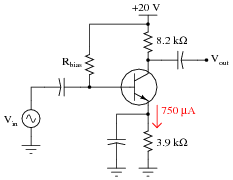AV
VC
• #### Question 18

Calculate the approximate voltage gain (AV) for the following common-emitter amplifier circuit, expressing it both as a ratio and as a figure in decibels. Also calculate the quiescent DC voltages measured at the three terminals of the transistor with respect to ground (VB, VE, and VC). Assume a silicon transistor:AV (as a ratio) ≈
AV (in decibels) ≈
VB
VE
VC
• #### Question 19

Predict how all transistor currents (IB, IC, and IE) and the output voltage signal will be affected as a result of the following faults. Consider each fault independently (i.e. one at a time, no multiple faults):Capacitor Cin fails open:
Solder bridge (short) past resistor R1:
Resistor R1 fails open:
Resistor RC fails open:
Resistor RE fails open:
Capacitor Cbypass fails shorted:

For each of these conditions, explain why the resulting effects will occur.

• #### Question 20

Complete the table of voltages and currents for several given values of input voltage in this common-base amplifier circuit. Assume that the transistor is a standard silicon NPN unit, with a nominal base-base junction forward voltage of 0.7 volts:VE VB IB IC VRC VC 0.0 V -0.5 V -0.8 V -1.0 V -1.1 V -1.2 V -1.3 V

Calculate the voltage gain of this circuit from the numerical values in the table:

$$A_V=\frac{\triangle V_{out}}{\triangle V_in}}=$$

• #### Question 21

Describe the functions of resistors R1 and R2, and capacitor C1, in this amplifier circuit. What purpose do they serve?Would it be possible for this amplifier circuit to operate in Class A mode without this voltage divider/capacitor network? Explain your answer.

• #### Question 22

Explain the effects of increasing R3’s resistance in this amplifier circuit. As R3 becomes more resistive, will the input signal (Vin) have more or less effect on the output voltage (Vout) than before? Express your answer in terms of voltage gain (AV).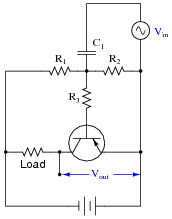• #### Question 23

Explain the effects of increasing the load resistance in this amplifier circuit. As the load becomes more resistive, will the input signal (Vin) have more or less effect on the output voltage (Vout) than before? Express your answer in terms of voltage gain (AV).• #### Question 24

Explain how you could measure the AC voltage gain of a functioning Class A amplifier circuit, as opposed to predicting its gain from known component values.

• #### Question 25

Identify the type of transistor amplifier configuration in these schematic diagrams as either common-emitter, common-collector, or common-base.• #### Question 26

Common-emitter, common-collector, and common-base amplifier circuits are sometimes referred to as grounded-emitter, grounded-collector, and grounded-base, respectively, because these configurations may actually be built with those respective terminals connected straight to ground.

Although this may not be very practical for ease of biasing, it can be done. Draw the rest of the circuit necessary to provide class-A operation for each of these (partial) transistor circuits. Be sure to show where the DC power source, signal input, and signal output connect:• #### Question 27

Define what a common-collector transistor amplifier circuit is. What distinguishes this amplifier configuration from the other single-BJT amplifier configurations, namely common-emitter and common-base?

Also, describe the typical gains (voltage and current) of this amplifier configuration, and whether it is inverting or noninverting.

• #### Question 28

Define what a common-emitter transistor amplifier circuit is. What distinguishes this amplifier configuration from the other single-BJT amplifier configurations, namely common-collector and common-base?

Also, describe the typical gains (voltage and current) of this amplifier configuration, and whether it is inverting or noninverting.

• #### Question 29

Define what a common-base transistor amplifier circuit is. What distinguishes this amplifier configuration from the other single-BJT amplifier configurations, namely common-collector and common-emitter?

Also, describe the typical gains (voltage and current) of this amplifier configuration, and whether it is inverting or noninverting.

• #### Question 30

Compared to common-collector and common-emitter amplifiers, common-base circuits have few practical applications. Explain why.

• #### Question 31

Predict how all transistor currents (IB, IC, and IE) and the output voltage signal will be affected as a result of the following faults. Consider each fault independently (i.e. one at a time, no multiple faults):Capacitor Cout fails open:
Solder bridge (short) past resistor R1:
Resistor R1 fails open:
Resistor RC fails open:
Resistor RE fails open:
Capacitor Cbypass fails shorted:

For each of these conditions, explain why the resulting effects will occur.

• #### Question 32

The voltage gain of this amplifier circuit, unlike other amplifier configurations, is completely independent of the load resistor value:No matter what the resistance of the load, the amplifier’s voltage gain remains the same. Explain why this is so.

• #### Question 33

Temperature changes are well known to affect transistor operation. For instance, if we were to apply a constant voltage between the base and emitter of a transistor and increase its temperature over time, the collector current would increase:First, describe why the collector current changes, if the input voltage is held constant. Then, determine the relative degree of output voltage change (∆Vout) resulting from this thermal effect in the following two amplifier circuits:What is different in the responses of these two circuits to temperature changes? Why does one circuit respond so much differently than the other?

If both these amplifier circuits had AC signal inputs, and were biased for Class A operation, what effect would an increase in temperature have on each of them? State your answer in terms of AC voltage gain and Q-point.• #### Question 34

One major different between a common-emitter amplifier configuration and a common-collector amplifier configuration is a principle called negative feedback, where changes in output voltage “feed back” to influence the amplifier’s input signal, which in turn influences the output voltage again. Common-collector amplifier circuits have large amounts of negative feedback inherent to their design.

The absence or presence of negative feedback in an amplifier circuit has profound effects on voltage gain (AV). Compare the relative voltage gains of the following amplifiers:At first, the low voltage gain of the common-collector amplifier may appear to be a disadvantage of that circuit design. However, there is one major benefit relevant to the common-collector amplifier’s voltage gain, being a direct result of negative feedback. What is this advantage?

• #### Question 35

One way to reap the advantages of negative feedback enjoyed in common-collector amplifier circuits, in a common-emitter amplifier, is to add components that intentionally “feed back” some of the output signal to the transistor’s input in a degenerative fashion: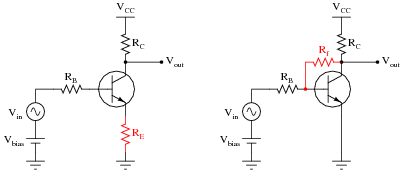Explain what the term negative (or degenerative) means with reference to feedback, and explain how each of these techniques works to produce this type of feedback. Also, explain one disadvantage of applying negative feedback to a common-emitter amplifier circuit.

• #### Question 36

Sometimes a feedback network is purposely placed in an amplifier circuit, like the Rf-Cf combination shown in the following schematic: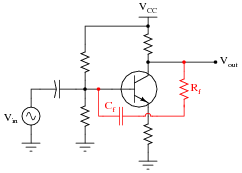Explain what will happen to the amplifier circuit’s performance if either one of the components in this feedback network fails open.

• #### Question 37

Amplifier distortion occurs when its gain varies as a function of the instantaneous signal amplitude. That is, some parts of the signal waveform become amplified more than others, and this results in the waveform taking on a slightly different shape.

All active devices, bipolar junction transistors included, are nonlinear to some extent. This term means that their gain varies throughout their operating ranges. During the 1920’s, an electrical engineer named Harold Black was pondering this problem in the design of telephone system amplifiers. His solution came to him in a flash of insight one day, as he was commuting from work on a ferry boat. Explain what his solution to this problem was.

• #### Question 38

A popular method of “reclaiming” some of the lost voltage gain resulting from the addition of an emitter resistor (RE) to a common-emitter amplifier circuit is to connect a “bypass” capacitor in parallel with that resistor: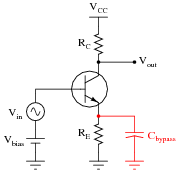Explain why this technique works to increase the circuit’s AC voltage gain, without leading to the problems associated with directly grounding the emitter.

• #### Question 39

Some common-emitter amplifier circuits use partial bypassing of emitter resistance, with the bypass capacitor connected in parallel with only one of two series resistors:Explain the purpose of this arrangement. How does this differ in performance from the simple one-resistor emitter feedback design, or a grounded-emitter amplifier with no emitter resistor at all?

• #### Question 40

A student builds this common-emitter amplifier so they he may amplify the audio signals from a microphone to power a speaker:Unfortunately, the results are considerably less than expected: although some sound does come out of the speaker, it is not enough to be considered a success. Another student inspects the design and cryptically mumbles something about “poor impedance matching,” leaving the first student somewhat confused.

Explain what impedance matching means in this context, where the mis-match might be in this circuit, and what might be done to correct it.

• #### Question 41

Each of the following faults will cause this audio amplifier circuit to stop working. Determine what diagnostic voltage measurement(s) would positively identify each one of the faults.Microphone coil fails open:
Capacitor C1 fails shorted:
Resistor R1 fails open:
Resistor R2 fails open:
Capacitor C3 fails open:
Transformer T1 primary winding fails open:
• #### Question 42

Suppose this microphone amplifier circuit used to function fine, but now has stopped outputting any sound at all:Initial diagnostic measurements show all quiescent (DC) voltages to be normal. From this data, where would you suspect the problem is, and where would you suspect the problem is not?

• #### Question 43

Sometimes you will see amplifier circuits expressed as collections of impedances and dependent sources: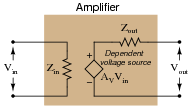With this model, the amplifier appears as a load (Zin) to whatever signal source its input is connected to, boosts that input voltage by the gain factor (AV), then outputs the boosted signal through a series output impedance (Zout) to whatever load is connected to the output terminals: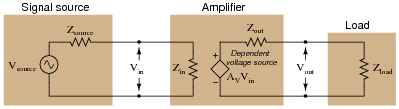Explain why all these impedances (shown as resistors) are significant to us as we seek to apply amplifier circuits to practical applications. Which of these impedances do you suppose are typically easier for us to change, if they require changing at all?

• #### Question 44

Complete the table of output voltages, output currents, and input currents for several given values of input voltage in this common-collector amplifier circuit. Assume that the transistor is a standard silicon NPN unit, with a nominal base-emitter junction forward voltage of 0.7 volts:Vin Vout Iin Iout 0.8 V 1.5 V 3.0 V 4.5 V 6.0 V 7.5 V

Calculate the amount of impedance “seen” by the input voltage source Vin, given the following definition for impedance:

$$Z_{in}=\frac{\triangle V_{in}}{\triangle I_{in}}=$$

• #### Question 45

Calculate the approximate input impedance (Zin) of this amplifier circuit:Also, explain why input impedance is an important factor in amplifier circuits.

• #### Question 46

The voltage divider network employed to create a DC bias voltage for many transistor amplifier circuits has its own effect on amplifier input impedance. Without considering the presence of the transistor or the emitter resistance, calculate the impedance as “seen” from the input terminal resulting from the two resistors R1 and R2 in the following common-collector amplifier circuit: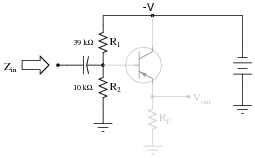Remember, what you are doing here is actually determining the Thévenin/Norton equivalent resistance as seen from the input terminal by an AC signal. The input coupling capacitor reactance is generally small enough to be safely ignored.

Next, calculate the input impedance of the same circuit, this time considering the presence of the transistor and emitter resistor, assuming a current gain (β or hfe) of 60, and the following formula for impedance at the base resulting from β and RE:

$$Z_B \approx (\beta+1)R_E$$Develop an equation from the steps you take in calculating this impedance value.

• #### Question 47

The voltage divider network employed to create a DC bias voltage for many transistor amplifier circuits has its own effect on amplifier input impedance. Without considering the presence of the transistor or the emitter resistance, calculate the impedance as “seen” from the input terminal resulting from the two resistors R1 and R2 in the following common-collector amplifier circuit: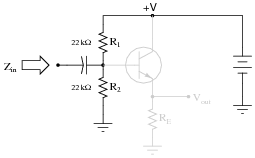Remember, what you are doing here is actually determining the Thévenin/Norton equivalent resistance as seen from the input terminal by an AC signal (consider the coupling capacitor reactance to be negligibly small).

Next, calculate the input impedance of the same circuit, this time considering the presence of the transistor and emitter resistor, assuming a current gain (β or hfe) of 42:Develop an equation from the steps you take in calculating this impedance value.

• #### Question 48

Determining the output impedance of a common-emitter amplifier is impossible unless we know how to model the transistor in terms of components whose behavior is simple to express.When in its active mode, a transistor operates like a current regulator. This is similar enough to the behavior of a current source that we may use a source to model the transistor’s behavior for the sake of this impedance determination: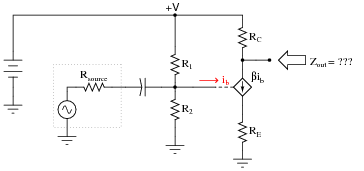Now, apply the same steps you would use in determining the Thévenin or Norton equivalent impedance to the output of this amplifier circuit, and this will yield the amplifier’s output impedance. Draw an equivalent circuit for the amplifier during this Thévenizing/Nortonizing process to show how the output impedance is determined.

• #### Question 49

What is the ideal amount of load impedance for this amplifier circuit, so that maximum power will be delivered to it?Suppose we wished to drive an 8 ohm audio speaker with this amplifier circuit. How could we better match the amplifier’s impedance to the speaker’s?

• #### Question 50

Calculate the ideal amount of load impedance for this amplifier circuit, so that maximum power will be delivered to it:• #### Question 51

Explain each of the mathematical approximations for this typical common-collector amplifier circuit:$$A_V \approx 1$$

$$Z_{in} \approx R_1 || R_2 || (\beta +1)[r’_e+(R_E|| R_{load})]$$

$$Z_{out} \approx R_E ||(r’_e+\frac{R_1||R_2||R_{source}}{\beta+1})$$

What does each term in each expression represent, and why do they relate to one another as shown?

• #### Question 52

In a common-collector transistor amplifier circuit with voltage divider biasing, the input impedance (Zin) is a function of load impedance, emitter resistance (RE), and the two biasing resistances (R1 and R2). Often, the biasing resistances are of sufficiently low value to swamp the input impedance of the transistor, so that R1 and R2 constitute the heaviest load for any input signals driving the amplifier.$$Z_{in} \approx R_1 || R_2 || (\beta +1)[r’_e+(R_E||R_{load})]$$

This is a shame, because the only practical purpose served by R1 and R2 is to provide a stable bias voltage so the transistor always functions in class A mode. In order to provide a stable bias, these resistors have to be relatively low in value compared to the impedance seen at the base of the transistor (resulting from the load). Otherwise, changes in dynamic emitter resistance (r′e) could result in significant bias shifts. So, the naturally high input impedance of the common-collector transistor configuration is spoiled by the necessary presence of R1 and R2.

A clever way to recover some of that naturally large input impedance is to add a bit of regenerative (positive) feedback to the circuit in the form of a capacitor and another resistor. This technique is given an equally clever name: bootstrapping.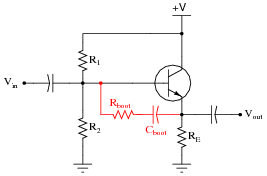Explain how bootstrapping works, and why that particular name is given to the technique.

• #### Question 53

A common set of equations for calculating input and output impedances of common-collector amplifier circuits is as follows:

$$Z_{in} \approx R_1 || R_2 || (\beta +1)[r’_e+(R_E||R_{load})]$$

$$Z_{out} \approx R_E || (r’_e+\frac{R_1||R_2||R_{source}}{\beta +1})$$

If precision is not required, we may greatly simplify these equations by assuming the transistor to be ideal; i.e. having an infinite current gain (β = ∞). Re-write these equations accordingly, and explain how you simplified each one.

• #### Question 54

Approximate the following values for this common-collector amplifier circuit, assuming the use of a silicon transistor:AV (as a ratio) ≈
AV (in decibels) ≈
Zin
Zout
• #### Question 55

Explain each of the mathematical approximations for this typical common-emitter amplifier circuit (with a bypass capacitor):$$A_V \approx \frac{R_C||R_{load}}{r'_e}$$

$$Z_{in} \approx R_1 || R_2 || (\beta+1)r’_e$$

$$Z_{out} \approx R_C$$

What does each term in each expression represent, and why do they relate to one another as shown?

• #### Question 56

Explain each of the mathematical approximations for this typical common-emitter amplifier circuit (with the dynamic emitter resistance ßwamped” by RE):$$A_V \approx \frac{R_C||R_{load}}{r'_e+R_E}$$

$$Z_{in} \approx R_1||R_2||(\beta+1)(r’_e+R_E)$$

$$Z_{out} \approx R_C$$

What does each term in each expression represent, and why do they relate to one another as shown?

• #### Question 57

A common set of equations for calculating input and output impedances of bypassed common-emitter amplifier circuits is as follows:

$$Z_{in} \approx R_1||R_2||(\beta+1)r’_e$$

$$Z_{out} \approx R_C$$

If precision is not required, we may greatly simplify the first equation by assuming the transistor to be ideal; i.e. having an infinite current gain (β = ∞). Re-write the first equation accordingly, and explain how you simplified it.

• #### Question 58

Approximate the following values for this common-emitter amplifier circuit, assuming the use of a silicon transistor: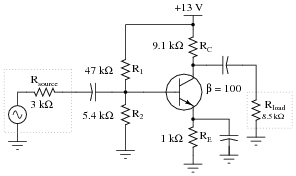AV (as a ratio) ≈
AV (in decibels) ≈
Zin
Zout
• #### Question 59

A parasitic property of semiconductor PN junctions is capacitance across the depletion regions. This is often referred to as the Miller Effect. In transistor circuits, the Miller effect contributes to a decrease in voltage gain as signal frequency increases.

Explain why junction capacitances make the voltage gain of an amplifier decrease with increasing frequency.

• #### Question 60

The BJT amplifier configuration most affected by the Miller effect at high frequencies is the common-emitter. Common-collector and common-base amplifier configurations do not suffer the same great losses of voltage gain at high frequency as the common-emitter circuit does. After examining the following amplifier circuits (with the Miller effect capacitance shown external to the transistors), explain why: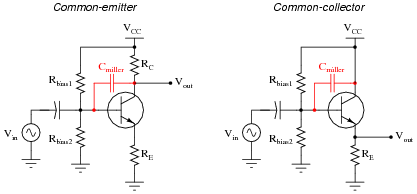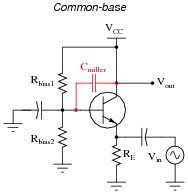• #### Question 61

The “Miller capacitance” of a transistor in a common-emitter configuration is often expressed as the product of the transistor’s base-to-collector junction capacitance (CBC) and $$\beta +1$$:

$$C_{miller}=C_{BC}(\beta +1)$$

Why is this? What purpose does it serve to include the transistor’s gain into the calculation, rather than just expressing the junction capacitance as it is?